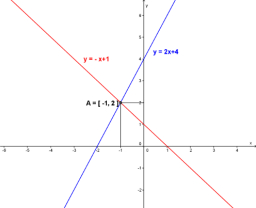The sum

The sum of two numbers is 69 and the difference is 11. Which numbers are they?

a =  40
b =  29

Step-by-step explanation:

a+b=69
a-b=11

a+b = 69
a-b = 11

a = 40
b = 29

Our linear equations calculator calculates it.Did you find an error or inaccuracy? Feel free to write us. Thank you!Tips to related online calculators
Do you have a system of equations and looking for calculator system of linear equations?